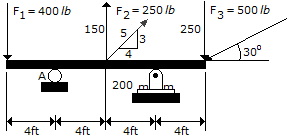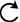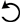# Engineering Mechanics - Force System Resultants - Discussion

### Discussion :: Force System Resultants - General Questions (Q.No.2)

2.Determine the moment of force F1 about point A on the beam.

 [A]. M1 = 1600 ft-lb[B]. M1 = 100 ft-lb[C]. M1 = 100 ft-lb[D]. M1 = 1600 ft-lbExplanation:

No answer description available for this question.

 Karthik said: (Apr 8, 2012) Simply we know the M=F*D then 400*horizontal distance (4) =1600 anticlockwise moment.

 Kusha Kumar.Poloju said: (Dec 26, 2012) * {r_{OF}} is the vector from point A to the position where quantity F1 is applied. * represents the cross product of the vectors. Therefore, M1=force(F1)*perpendicular distance(4ft), M1=400lb*4ft, M1=1600ft-lb. And the direction is getting from right hand screw principle as anti-clockwise direction.

 Albert Villaganas said: (Jul 7, 2013) Do you have a deeper explanation and solution regarding on this problem?

 Mayuresh Date said: (Jul 24, 2013) According to fundamental principles, we can apply a balance force system anywhere along the rigid body. So if we apply at point A a balance force system of 400lb vertically upward and downward, they will cancel out each other and net effect remains the same but the force F1 will form a couple with the vertical 400lb force at A. The moment of a couple is nothing but the product of one of the force of couple and its couple arm which is here 4ft, Since the couple is a vector qty, hence we will get the answer as 400*4=1600lb-ft in counterclockwise direction.

 Md Mahfooz said: (Sep 30, 2014) How can I derive moment?

 Ftanegest said: (Dec 9, 2015) It is well. But it needs more clarifications.

 Atchuth said: (Aug 14, 2018) Moment=force * distance. By observing the beam we have one force on the left side, apply moment formula. F1* 4=m(A). 400 * 4==1600.